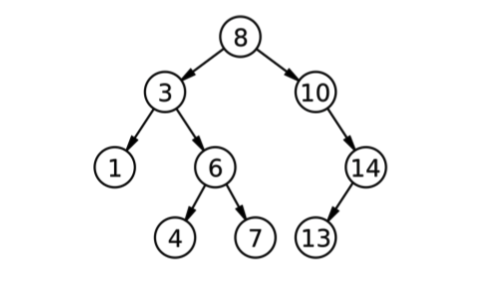# 12.2: Binary search tree

A binary search tree (BST) is a tree where each node contains a key, and every node has the “BST property”:

1. If node has a left child, all keys in the left subtree must be less than the key in node.
2. If node has a right child, all keys in the right subtree must be greater than the key in node.

Figure $$\PageIndex{1}$$ shows a tree of integers that has this property. This figure is from the Wikipedia page on binary search trees at http://thinkdast.com/bst, which you might find useful while you work on this exercise.Figure $$\PageIndex{1}$$: Example of a binary search tree.

The key in the root is 8, and you can confirm that all keys to the left of the root are less than 8, and all keys to the right are greater. You can also check that the other nodes have this property.

Looking up a key in a binary search tree is fast because we don’t have to search the entire tree. Starting at the root, we can use the following algorithm:

1. Compare the key you are looking for, target, to the key in the current node. If they are equal, you are done.
2. If target is less than the current key, search the left tree. If there isn’t one, target is not in the tree.
3. If target is greater than the current key, search the right tree. If there isn’t one, target is not in the tree.

At each level of the tree, you only have to search one child. For example, if you look for target = 4 in the previous diagram, you start at the root, which contains the key 8. Because target is less than 8, you go left. Because target is greater than 3 you go right. Because target is less than 6, you go left. And then you find the key you are looking for.

In this example, it takes four comparisons to find the target, even though the tree contains nine keys. In general, the number of comparisons is proportional to the height of the tree, not the number of keys in the tree.

So what can we say about the relationship between the height of the tree, h, and the number of nodes, n? Starting small and working up:

• If h=1, the tree only contains one node, so n=1.
• If h=2, we can add two more nodes, for a total of n=3.
• If h=3, we can add up to four more nodes, for a total of n=7.
• If h=4, we can add up to eight more nodes, for a total of n=15.

By now you might see the pattern. If we number the levels of the tree from 1 to h, the level with index i can have up to $$2^{i-1}$$ nodes. And the total number of nodes in h levels is $$2^h -1$$. If we have

$n = 2^h -1 \nonumber$

we can take the logarithm base 2 of both sides:

$\log_{2}{n} \approx h \nonumber$
which means that the height of the tree is proportional to $$\log{n}$$, if the tree is full; that is, if each level contains the maximum number of nodes.

So we expect that we can look up a key in a binary search tree in time proportional to $$\log{n}$$. This is true if the tree is full, and even if the tree is only partially full. But it is not always true, as we will see.

An algorithm that takes time proportional to $$\log{n}$$ is called “logarithmic” or “log time”, and it belongs to the order of growth $$O(\log{n})$$.